CLAT  >  Data Interpretation

# Data Interpretation - Quantitative Techniques for CLAT

## Introduction

Data Interpretation is the section that consists of charts and tables, myriad of graphs, from which candidates will have to analyze and glean data.

The data interpretation questions may seem lengthy but the key to solving these questions is to identify the important pieces of data and approach the question in a step-by-step process. Charts, Venn diagrams, myriad graphs, tables, etc. usually dominate the data interpretation section.

### How to solve Data Interpretation questions

• Read the questions carefully and understand the data before you proceed to solve the questions
• There might be unnecessary data given in the table or graphs to confuse you. So, make sure to cross-check the answer.
• Ensure to check the units before marking the answer.
• Check if the data is given in percentage or decimal as both have different calculations.

### Types of Data Interpretation Questions

• Tabular
• Bar chart

Example 1:

Number of Employees of various departments of Parle India for the year 2011 to 2017 is given. Answer the questions based on the data provided.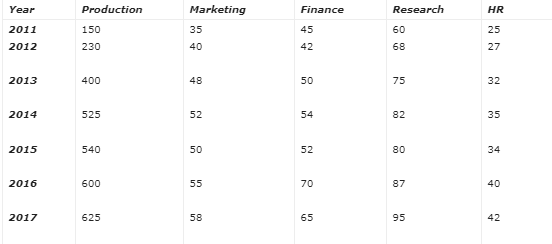1. In which year the number of employees was 140% more than the no. of employees in 2011.

(a) 2013

(b) 2014

(c) 2015

(d) 2016

The correct answer is option (c).

Solution: Look at the options first, 4 options for the answer are: 2013, 2014, 2015 and 2016.

As we need the total no of employees, in each department, in question paper only after the last column you can write down the sum of no. of employees.

Now, an increase of 140% in no. of employees of 2011, i.e., as per increase in p% concept we can write as x(1+140/100)=x(2.14).

Here, x is the total number of employees in the year 2011, x= 315 therefore 140% increase in 315 will be 756 i.e., no. of employees in the year 2015

2. In how many years, the number of employees in the production department is more than 50 % of total employees?

(a) 2

(b) 5

(c) 4

(d) 6

The correct answer is option (d).

Solution:

As in the last question you would have calculated the total number of employees in different years, this question can be solved orally by just looking at numbers. More than 50% would require you to just calculate 50% of the total employees, for example, 315/2=102(approx.), 407/2=203(approx.) while no of employees in 2012 in the production dept. is 230 hence more than 50% similarly calculate for other years.

In whichever year you find the number of employees in the production department is more than 50% make a tick at one side and check further you will find that out 7 years, except 2011 in all other years no. of employees in the production department is 50% more than total no. of employees.

Alternative: Calculation based solution

Percentage of employees of production department wrt to total employees:

• In 2011 : 150*100/315 = 47.6%
• In 2012: 230*100/407 = 56.51%
• In 2013: 400*100/605 = 66.11%
• In 2014: 525*100/748 = 70.18%
• In 2015: 540*100/756 = 71.4%
• In 2016: 600*100/852 =70.42%
• In 2017: 625*100/885=70.62%

Except 2011 in all other years the percentage of production department employees is more than 50%.

Question for Data Interpretation
Try yourself:Study the following table and answer the questions based on it.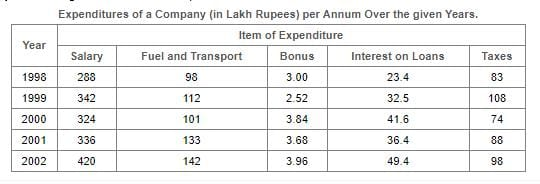What is the average amount of interest per year which the company had to pay during this period?

Question for Data Interpretation
Try yourself:Total expenditure on all these items in 1998 was approximately what percent of the total expenditure in 2002?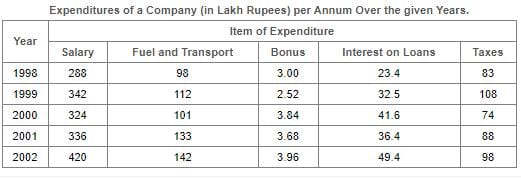Example 2: The bar graph given below shows the sales of books (in thousand number) from six branches of a publishing company during two consecutive years 2000 and 2001.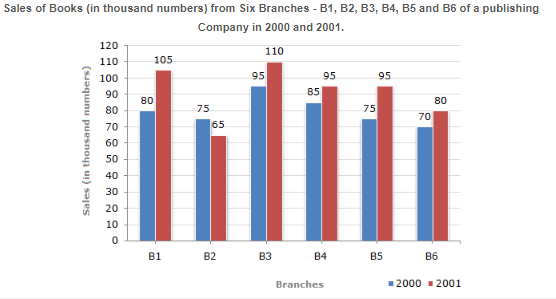1. What is the ratio of the total sales of branch B2 for both years to the total sales of branch B4 for both years?

(a) 2:3

(b) 3:5

(c) 4:5

(d) 7:9

The correct answer is option (d).

Explanation: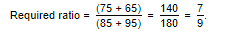2. Total sales of branch B6 for both the years is what percent of the total sales of branches B3 for both the years?

(a) 68.54%

(b) 71.11%

(c) 73.17%

(d) 75.55%

The correct answer is option (c).

Explanation:Question for Data Interpretation
Try yourself:The ratio of the number of years, in which the foreign exchange reserves are above the average reserves, to those in which the reserves are below the average reserves is?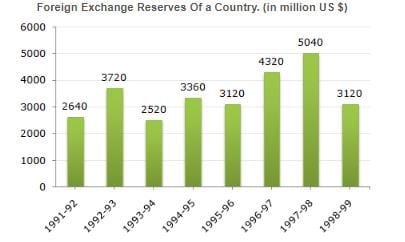Question for Data Interpretation
Try yourself:For which year, the percent increase of foreign exchange reserves over the previous year, is the highest?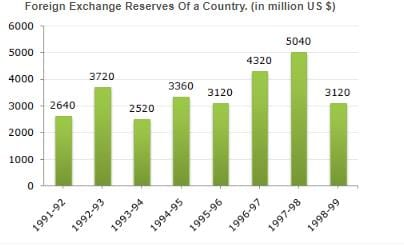The document Data Interpretation | Quantitative Techniques for CLAT is a part of the CLAT Course Quantitative Techniques for CLAT.
All you need of CLAT at this link: CLAT

## FAQs on Data Interpretation - Quantitative Techniques for CLAT

 1. What is data interpretation?Ans. Data interpretation is the process of analyzing and making sense of data by examining patterns, relationships, and trends to derive meaningful insights and draw conclusions.
 2. Why is data interpretation important?Ans. Data interpretation is crucial because it helps in making informed decisions, identifying opportunities, and solving complex problems. It allows organizations to uncover hidden patterns and trends in data, which can lead to better strategies and improved performance.
 3. What are the steps involved in data interpretation?Ans. The steps involved in data interpretation include data collection, data cleaning and preprocessing, data analysis, data visualization, and drawing conclusions. These steps help in transforming raw data into meaningful information.
 4. How can data interpretation be used in business?Ans. Data interpretation is widely used in business for various purposes. It can help in market research, customer segmentation, demand forecasting, identifying sales trends, optimizing supply chain operations, and improving overall business performance.
 5. What are some common challenges in data interpretation?Ans. Some common challenges in data interpretation include dealing with large volumes of data, ensuring data quality and accuracy, handling missing or incomplete data, choosing the right data analysis techniques, and effectively communicating the findings to stakeholders.

## Quantitative Techniques for CLAT

55 videos|35 docs|98 tests

## Quantitative Techniques for CLAT

55 videos|35 docs|98 tests
Signup to see your scores go up within 7 days! Learn & Practice with 1000+ FREE Notes, Videos & Tests.
10M+ students study on EduRev
Track your progress, build streaks, highlight & save important lessons and more!(Scan QR code)
Related Searches

,

,

,

,

,

,

,

,

,

,

,

,

,

,

,

,

,

,

,

,

,

;